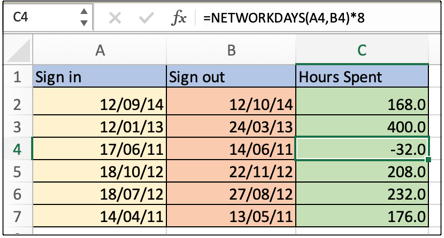# How to Calculate the Number of Weekday Hours Between Two Dates in ExcelIn this article, we will learn How to Calculate the Number of Weekday Hours Between Two Dates in Excel.

Scenario:

Usually Saturdays and Sundays are weekends meaning non working days or non working hours. Sometimes calculating the total work hours between two dates,  we can manually calculate the number of days between the days and subtract the number of days and multiply the result with 24 to get hours. This doesn't look easy and can lead to mistakes if the two given dates are too much apart. NETWORKDAYS function has the fixed type of weekend which is Saturday and Sunday. So now we learn the extended version of WORKDAYS function which lets you edit the weekends as per choice.

Number of weekday hours formula in Excel

To get the networking days between dates having holidays use NETWORKDAYS function in Excel. It takes Saturday and Sunday as default holidays.

NETWORKDAYS Function Syntax:

 =NETWORKDAYS (start , end , holidays ) * hours

Start_date : count of first date

End_date : count of end date

Holidays : [optional] argument in the function

hours : work hours per day

Example :

All of these might be confusing to understand. Let's understand how to use the function using an example. Here we have some sample dates where we need to find the total working hours between the two given dates where Saturday and Sunday are non working days and considering no national holidays as working days. Here we have a sign in date, sign out date.

Use the formula:

 =NETWORKDAYS(A4,B4)*8As you can see, formula returns weekdays or working hours in excel. You must be wondering why there is a negative hours, and it can't be so. It means the start date is after the end date. Negative value also finds out the defaulter here.

Here are all the observational notes using the formula in Excel
Notes :

1. Excel stores dates as serial numbers and is used in calculation by the function. So it’s recommended to use dates as cell reference or using the DATE function instead of giving direct argument to the function. Check the validation of date values if the function returns #VALUE! Error.
2. If the start date is greater than the end date, the function returns the number of days with a negative sign.
3. If the start date and end date is the same date and that date is working, the function returns 1.
4.  If the start date or end date is out of range, then function returns #NUM! Error.
5. Holidays argument must be given as array reference, not the individual dates separated using commas, the function throws too many arguments error.

Hope this article about How to Calculate the Number of Weekday Hours Between Two Dates in Excel is explanatory. Find more articles on calculating values and related Excel formulas here. If you liked our blogs, share it with your friends on Facebook. And also you can follow us on Twitter and Facebook. We would love to hear from you, do let us know how we can improve, complement or innovate our work and make it better for you. Write to us at info@exceltip.com.

Related Articles :

How to use the NETWORKDAYS function in Excel : Returns the working days between the two given dates using the NETWORKDAYS function.

How to use the WORKDAYS function in Excel : Returns a workday date value after days added to the start_date using the WORKDAYS function in Excel.

Count holidays between dates in Excel : count non working days between the two given dates using the DATEDIF and NETWORKDAYS function in Excel.

Extract days between dates ignoring years in Excel : count the days between the two dates using different functions and mathematical operation in Excel

Count Birth Dates By Month in Excel : count the number of dates lying in a particular month using the SUMPRODUCT and MONTH function in Excel.

SUM price by weekdays in Excel : sum price values corresponding to the number of dates in a particular weekday using the SUMPRODUCT and WEEKDAY function in Excel.

Popular Articles :